#### How to Reference a Cell in Excel Vba

In this article, we are going to learn how to refer to a cell in VBA. When using one of the most common task is to refer to a cell or a range of cell and then do something to it like changing the format of the cell or entering a formula in the cell. An example is highlighting the cell A1.

## Basics

You can usually do this in one statement that identifies the range and also changes a property or applies a method. A Range object in Visual Basic can be either a single cell or a range of different cells.

Two methods to refer to a cell in VBA are: the range method and the cells method. These method are further discussed down below.

The Range Method:

This method allows you to refer to a Cell in VBA by using its address. I.e. Range (“A1”) This is simple and useful in many situations.

The Cells Method: This method allows you to select a Cell by defining its numeric coordinates (row, column). I.e. Cells (1, 1) would refer to the cell in the first row of the first column, or range A1 In the following example we will use cell reference (by both the range method and cells method) to enter text in the cells.

## The ribbon

Right click anywhere on the ribbon and select “Customize the Ribbon” from the drop down menu.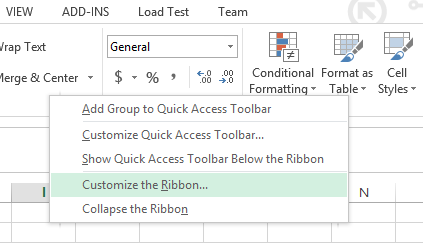An option dialog box will open. Click on the DEVELOPER check box (it is under “Customize the Ribbon Main Tabs”) and press ok.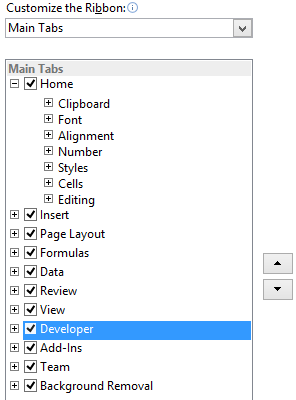The developer tap is now visible and is present next to the view tab on the top menu bar. Click on Developer tab and select “View Code”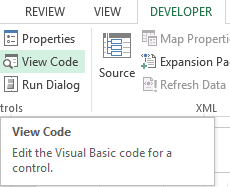## The code

A new window (Visual Basic Editor) will open which would have a dialog box in the center. You will write the code in the dialog box.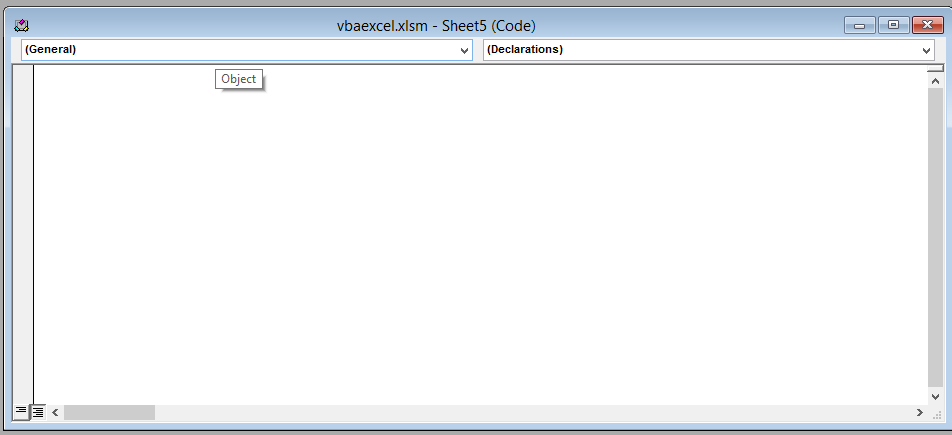Write the following line of code in the dialog box.

```Sub test ()
Range ("A1") = "HELLO"
Cells (2, 2) = "HOW"
Range ("C3") = "ARE"
Cells (4, 4) = "YOU"
Range ("E5") = "DOING"
Cells (6, 6) = "?"
Range ("B12").Select
End Sub```

After writing the code close the window by clicking on the cross(x) icon on the upper right side of the screen. Don’t worry. Excel won’t close.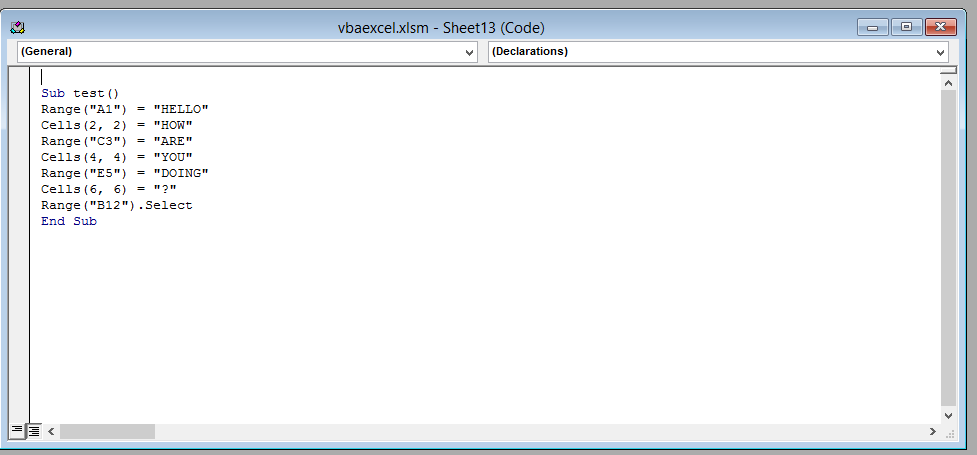Explanation: The code enter text into the cells using both the range method and the cells method. The second line of code (Range (“A1”) =”Hello) causes the text “HELLO” to be entered in cell A1 using the range method. The third line of code enters the text “HOW” in cell B2 using the cells method.

This will be the result. That’s it you have now successfully refer to a cell using VBA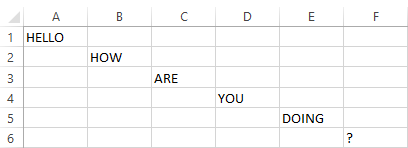## Key Takeaways

There are two methods to refer to a cell in VBA: the range method and the cells method.

The range method allows you to refer to a cell by its address, while the cells method allows you to refer to a cell by its numeric coordinates.

To use the range method, you can use the Range() function. For example, Range(“A1”) would refer to the cell in the first row of the first column.

See also  How to Assign a Macro to a Button in Excel

To use the cells method, you can use the Cells() function. For example, Cells(1, 1) would refer to the cell in the first row of the first column.

You can use cell references in VBA to enter text, formulas, and other data into cells.

## FAQ

Q: What is the difference between the range method and the cells method?

A: The main difference between the range method and the cells method is that the range method allows you to refer to a cell by its address, while the cells method allows you to refer to a cell by its numeric coordinates.

Q: When should I use the range method and when should I use the cells method?

A: The range method is usually used when you want to refer to a cell by its address. For example, if you want to enter the text “Hello” into cell A1, you would use the range method. The cells method is usually used when you want to refer to a cell by its numeric coordinates. For example, if you want to enter the text “Hello” into the cell in the first row of the first column, you would use the cells method.

Q: What are some other ways to refer to a cell in VBA?

A: You can also refer to a cell by its name. For example, if you have named cell A1 “MyCell”, you could refer to it as MyCell. You can also refer to a cell by its index. For example, if cell A1 is the first cell in a range, you could refer to it as Cells(1).